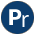#ordsets.pl -- Ordered set manipulation

Ordered sets are lists with unique elements sorted to the standard order of terms (see sort/2). Exploiting ordering, many of the set operations can be expressed in order N rather than N^2 when dealing with unordered sets that may contain duplicates. The library(ordsets) is available in a number of Prolog implementations. Our predicates are designed to be compatible with common practice in the Prolog community. The implementation is incomplete and relies partly on library(oset), an older ordered set library distributed with SWI-Prolog. New applications are advised to use library(ordsets).

Some of these predicates match directly to corresponding list operations. It is advised to use the versions from this library to make clear you are operating on ordered sets. An exception is member/2. See ord_memberchk/2.

The ordsets library is based on the standard order of terms. This implies it can handle all Prolog terms, including variables. Note however, that the ordering is not stable if a term inside the set is further instantiated. Also note that variable ordering changes if variables in the set are unified with each other or a variable in the set is unified with a variable that is `older' than the newest variable in the set. In practice, this implies that it is allowed to use `member(X, OrdSet)` on an ordered set that holds variables only if X is a fresh variable. In other cases one should cease using it as an ordset because the order it relies on may have been changed.

is_ordset(@Term) is semidet
True if Term is an ordered set. All predicates in this library expect ordered sets as input arguments. Failing to fullfil this assumption results in undefined behaviour. Typically, ordered sets are created by predicates from this library, sort/2 or setof/3.
ord_empty(?List) is semidet
True when List is the empty ordered set. Simply unifies list with the empty list. Not part of Quintus.
ord_seteq(+Set1, +Set2) is semidet
True if Set1 and Set2 have the same elements. As both are canonical sorted lists, this is the same as ==/2.
Compatibility
- sicstus
list_to_ord_set(+List, -OrdSet) is det
Transform a list into an ordered set. This is the same as sorting the list.
ord_intersect(+Set1, +Set2) is semidet
True if both ordered sets have a non-empty intersection.
ord_disjoint(+Set1, +Set2) is semidet
True if Set1 and Set2 have no common elements. This is the negation of ord_intersect/2.
ord_intersect(+Set1, +Set2, -Intersection)
Intersection holds the common elements of Set1 and Set2.
deprecated
- Use ord_intersection/3
ord_intersection(+PowerSet, -Intersection)
Intersection of a powerset. True when Intersection is an ordered set holding all elements common to all sets in PowerSet.
Compatibility
- sicstus
ord_intersection(+Set1, +Set2, -Intersection) is det
Intersection holds the common elements of Set1 and Set2. Uses ord_disjoint/2 if Intersection is bound to `[]` on entry.
ord_intersection(+Set1, +Set2, ?Intersection, ?Difference) is det
Intersection and difference between two ordered sets. Intersection is the intersection between Set1 and Set2, while Difference is defined by `ord_subtract(Set2, Set1, Difference)`.
- ord_intersection/3 and ord_subtract/3.
Insert an element into the set. This is the same as `ord_union(Set1, [Element], Set2)`.
ord_del_element(+Set, +Element, -NewSet) is det
Delete an element from an ordered set. This is the same as `ord_subtract(Set, [Element], NewSet)`.
ord_selectchk(+Item, ?Set1, ?Set2) is semidet
Selectchk/3, specialised for ordered sets. Is true when `select(Item, Set1, Set2)` and Set1, Set2 are both sorted lists without duplicates. This implementation is only expected to work for Item ground and either Set1 or Set2 ground. The "chk" suffix is meant to remind you of memberchk/2, which also expects its first argument to be ground. `ord_selectchk(X, S, T)` => `ord_memberchk(X, S)` & \+ `ord_memberchk(X, T)`.
author
- Richard O'Keefe
ord_memberchk(+Element, +OrdSet) is semidet
True if Element is a member of OrdSet, compared using ==. Note that enumerating elements of an ordered set can be done using member/2.

Some Prolog implementations also provide ord_member/2, with the same semantics as ord_memberchk/2. We believe that having a semidet ord_member/2 is unacceptably inconsistent with the *_chk convention. Portable code should use ord_memberchk/2 or member/2.

author
- Richard O'Keefe
ord_subset(+Sub, +Super) is semidet
Is true if all elements of Sub are in Super
ord_subtract(+InOSet, +NotInOSet, -Diff) is det
Diff is the set holding all elements of InOSet that are not in NotInOSet.
ord_union(+SetOfSets, -Union) is det
True if Union is the union of all elements in the superset SetOfSets. Each member of SetOfSets must be an ordered set, the sets need not be ordered in any way.
author
- Copied from YAP, probably originally by Richard O'Keefe.
ord_union(+Set1, +Set2, ?Union) is det
Union is the union of Set1 and Set2
ord_union(+Set1, +Set2, -Union, -New) is det
True iff `ord_union(Set1, Set2, Union)` and `ord_subtract(Set2, Set1, New)`.
ord_symdiff(+Set1, +Set2, ?Difference) is det
Is true when Difference is the symmetric difference of Set1 and Set2. I.e., Difference contains all elements that are not in the intersection of Set1 and Set2. The semantics is the same as the sequence below (but the actual implementation requires only a single scan).
```      ord_union(Set1, Set2, Union),
ord_intersection(Set1, Set2, Intersection),
ord_subtract(Union, Intersection, Difference).```

For example:

```?- ord_symdiff([1,2], [2,3], X).
X = [1,3].```